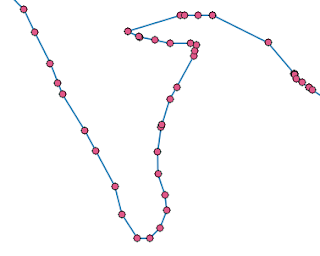# PyQGIS Tutorial: Geometry Conversion

Sometimes when doing a GIS task, we need to convert a vector file in a certain geometry to another geometry such as polygon to polyline, line to point, polyline to polygon, etc. There are some tools available in QGIS to do those conversions, we will access and use some of them using Python code.

### Polygon to Lines Conversion

Firstly let's start to convert polygon to polyline. To convert polygon to polyline we use QGIS polygontolines processing command. To run this command we just need an input file in polygon geometry and an output path to save the output file. The listing code below shows how to do it. Figure 1a shows a polygon before conversion, and figure 1b shows the result after conversion.

```import processing
input_layer='F:\lake_polygon.shp'
output_layer='F:\lake_lines.shp'
processing.run("qgis:polygonstolines", {'INPUT':input_layer,'OUTPUT':output_layer})
```Figure 1. Polygon to lines conversion. (a) Polygon before conversion. (b) Lines after conversion
How to do the reverse process, converting lines to polygon? For this operation we can use processing command linestopolygons, with the same two variables input file and output path.

### Convert Lines to Points

To convert line to point, the processing command from SAGA which is called convertlinestopoints is used. This command takes two parameters LINES and POINTS. LINES is input line to be converted and POINTS is output point. Figure 2 shows the result of line to point conversion. It can be seen that points will be created at each line's vertex.

```import processing
input_line='F:\lake_line.shp'
output_point='F:\lake_point.shp'
processing.run("saga:convertlinestopoints",{'LINES':input_line,'POINTS':output_point})
```Figure 2. Line to Point conversion output
Another option to convert line to point is using v.to.points command from GRASS. This command takes at least four parameters: input, output, use, and GRASS_OUTPUT_TYPE_PARAMETER. The use parameter can be 0 for node or 1 for vertex. So if 0 is given the command will create point at the node of the line, otherwise it will create point at each vertex of the line. GRASS_OUTPUT_TYPE_PARAMETER value usually is the same with use value. The command below is using to convert line to points at vertex which produce the same result as in figure 2.

```processing.run("grass7:v.to.points",{'input':input_line,'use':1,'output':output_point,'GRASS_OUTPUT_TYPE_PARAMETER':1})
```

### Point to Line Conversion

For point to line conversion the QGIS pointstopath command can be used to do this operation. As usual it needs input point and also output path to keep the result. Another mandatory parameter is ORDER_FIELD. This parameter will be used in the algorithm to define routing point. It means if a set of points that formed a curve line, but the order is not sequence, will produce an incorrect result. One more parameter that can be added is GROUP_FIELD. This parameter will grouped or segmented the output line based on a field value. In figure 3c can be seen the line output that grouped by two values in a field. In that figure can be seen that the two separate lines was created based on field value and they won't connecting to each other.

The following code was used to produce the result as in figure 3a. The next one is command that used GROUP_FIELD and produced result as in 3c.

```import processing
input_point='F:\input_point.shp'
output_line='F:\output_line.shp'
processing.run("qgis:pointstopath",{'INPUT':input_point,'ORDER_FIELD':'fid','OUTPUT':output_line})
```

```processing.run("qgis:pointstopath",{'INPUT':input_point,'ORDER_FIELD':'fid','GROUP_FIELD':'ZONE','OUTPUT':output_line})
```Figure 3. (a) Original point dataset. (b) Converted to single line. (c) Converted to two line segments

That's all the tutorial how to do geometry conversion such as converting polygon to lines, lines to point and point to lines. To learn more about QGIS Python programming. Visit QGIS Python Programming Series that offer other interesting topics.